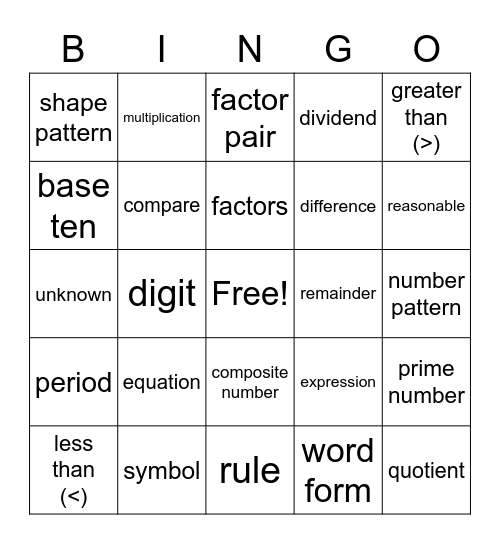# Unit 1, 2, and 3 Vocabulary Grade 4This bingo card has a free space and 34 words: value, word form, standard form, expanded form, period, compare, greater than (>), less than (<), multiplication, product, factors, factor pair, multiple, division, remainder, reasonable, symbol, unknown, equation, expression, composite number, prime number, shape pattern, number pattern, rule, quotient, dividend, divisor, difference, base ten, place value, digit, prime number and composite number.

⚠ This card has duplicate items: composite number (2), prime number (2)

## Play Online